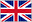# Week 3 Functions

0    15 tarjetas

términodefinicióndescribe a function from A to B.
A (total) function f from A to B, f: A->B, is a relation from A to B such that for all x ∈ A there is exactly one element in B, f(x) associated with x by a relation f
how is the expression f(x) read?
"f of x" or "f at x" or "f applied to x" also called the image of x
If f: A->B and f associates the element x ∈ A with the element y ∈ B what do we write?
f(x) = y or "f maps x to y ".
explain x ∈ A maps to y = f(x) ∈ B
A (total) function f maps a set of inputs (the set A) to the outputs (the set B)
what is the function f from N -> N that maps every natural number x to its cube x^3
f(x) =x^3
what is a partial function?
A partial function from A to B is like a function except that it might not be defined for some elements of A
Let f: A -> B (f is partial or total): What is the domain of f?
The subset D ⊆ A of all elements for which f is defined is called the domain of f. In case of a total function D=A. In case of a partial function, D ⊂ A
Let f: A->B(f is partial or total): what is the co-domain?
The set B is the co-domain of f
Let f: A->B(f is partial or total): what is the range of f?
range(f) = {f(x) |x ∈ A} or The range (image) of f, denoted by range(f), is the set of elements in the co-domain B that are associated with some element of A
when is a function such as f: A->B called injective?
A function f: A->B is called injective (also one-to-one) if it maps distinct elements ofA to distinct elements of B. or for all x, y ∈ A if x ≠y => f(x)≠f(y)
when is a function such as f: A->B called surjective?
A function f: A->B is called surjective (onto) if the range(f)is the co-domain B. To put it another way for all y∈B there exists x∈A such thatf(x)=y
when is a function such as f: A->B called bijective?
if it is both injective and surjective
Let f: A->B and g: B->C be functions. what is the composit of g with f
The composition of g with f is the function denoted by g ∘ f: A->C and denoted by(g∘f)(x) = g(f(x))for all x∈A